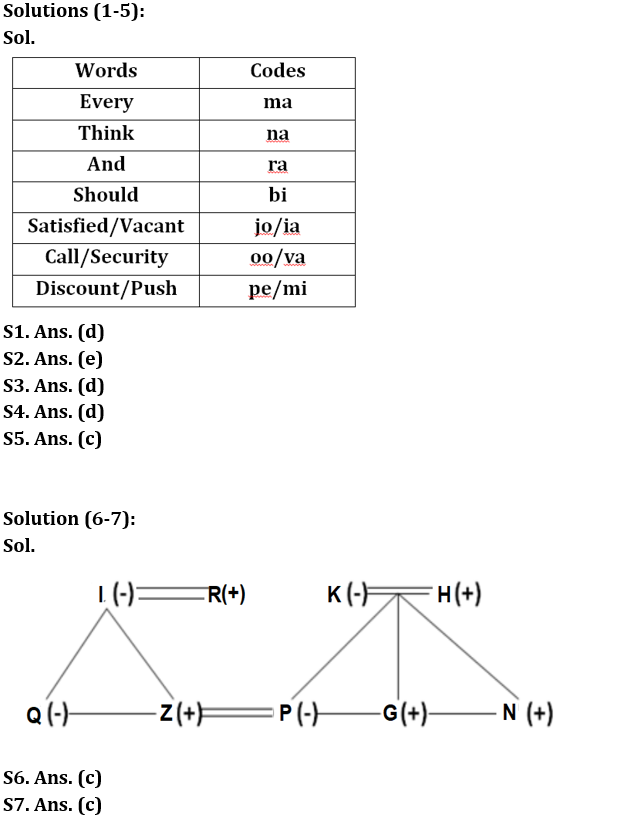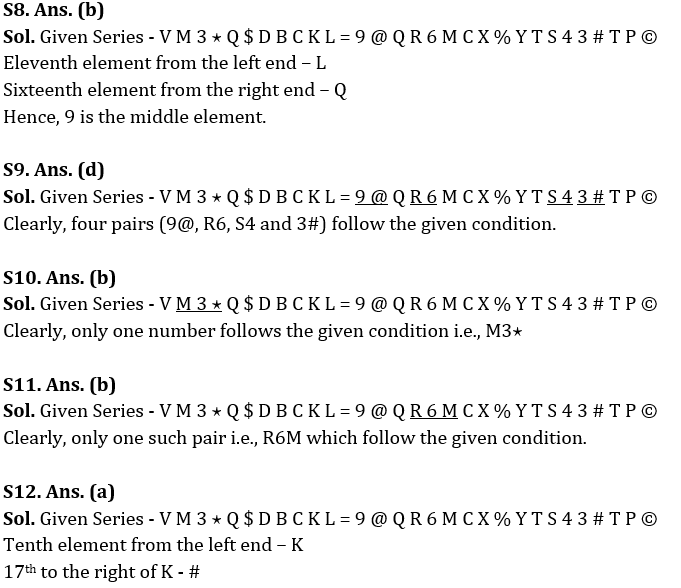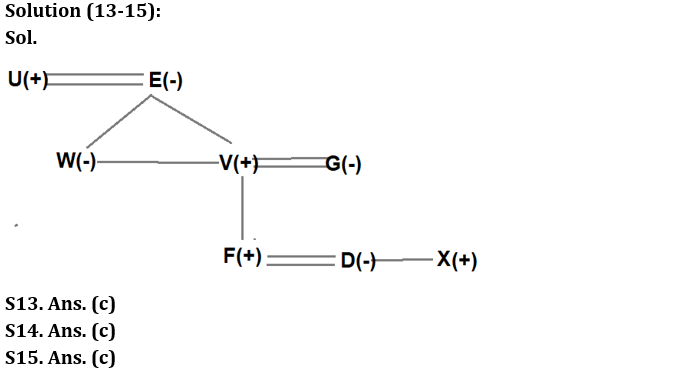Latest Banking jobs   »

# Reasoning Ability Quiz For IBPS RRB PO Prelims 2022- 21st June

Directions (1-5): Study the following information carefully and answer the questions accordingly.
In a certain code language,
‘Every satisfied vacant think’ is written as ‘ma na jo ia’,
‘Call and security every’ is written as ‘oo va ra ma’,
‘Think should discount push’ is written as ‘bi na pe mi’,
‘Call should security every’ is written as ‘bi oo ma va’.

Q1. What is the code for ‘and’?
(a) ma
(b) oo
(c) va
(d) ra
(e) None of these

Q2. What is the code for ‘security’?
(a) bi
(b) ra
(c) va
(d) oo
(e) Can’t be determined

Q3. What is the possible code for ‘push discount should’?
(a) mi ma na
(b) pe mi na
(c) mi bi ma
(d) pe mi bi
(e) None of these

Q4. What is the code for ‘should think?
(a) na va
(b) bi ma
(c) pe jo
(d) na bi
(e) None of these

Q5. What is the code for ‘every’?
(a) ra
(b) ia
(c) ma
(d) jo
(e) None of these

Directions (6-7): Study the following information carefully and answer the questions accordingly.
K is the mother of P who is the sister of G. P is married to Z. H is the parent of G. I is the mother of Z and Q is the only daughter of I. N and G are siblings. K has only one daughter. I is the wife of R.

Q6. How is Z related to N?
(a) Son
(b) Sister-in-law
(c) Brother-in-law
(d) Brother
(e) None of these

Q7. How is P related to I?
(a) Sister
(b) Daughter
(c) Daughter-in-law
(d) Mother
(e) Either Sister or Daughter

Directions (8-12): Study the following series carefully and answer the questions given below.

V M 3 ⋆ Q \$ D B C K L = 9 @ Q R 6 M C X % Y T S 4 3 # T P ©

Q8. Which of the following element is exactly in the middle of the eleventh element from the left end and sixteenth element from the right end in the above series?
(a) Q
(b) 9
(c) L
(d) P
(e) None of these

Q9. How many such numbers are there in the above series, each of which is either immediately preceded by a letter or immediately followed by a symbol but not both?
(a) One
(b) Two
(c) Three
(d) Four
(e) None of these

Q10. How many such numbers are there in the above series, each of which is immediately preceded by a letter and immediately followed by a symbol?
(a) None
(b) One
(c) Two
(d) Three
(e) None of these

Q11. How many such numbers are there, each of which is immediately preceded and immediately followed by a consonant?
(a) None
(b) One
(c) Two
(d) Three
(e) More than three

Q12. Which of the following element is seventeenth to the right of tenth element from the left end?
(a) #
(b) 3
(c) S
(d) T
(e) None of these

Directions (13-15): Read the following information carefully and answer the questions given below.
A three-generation family consists of eight members i.e., U, V, W, X, D, E, F and G in which three are married couples. V is the father of F. E is the mother of V. W is the sister-in-law of G. G is the mother-in-law of D. F is the grandson of U who is the father-in-law of G. X is the brother-in-law of F.

Q13. What is the relation of W with respect to U?
(a) Daughter-in-law
(b) Niece
(c) Daughter
(d) Granddaughter
(e) None of these

Q14. Who amongst the following is the grandmother of F?
(a) W
(b) G
(c) E
(d) D
(e) None of these

Q15. How many female members in the family?
(a) Five
(b) Three
(c) Four
(d) Can’t be determined
(e) None of the above

Solutions#### Congratulations!